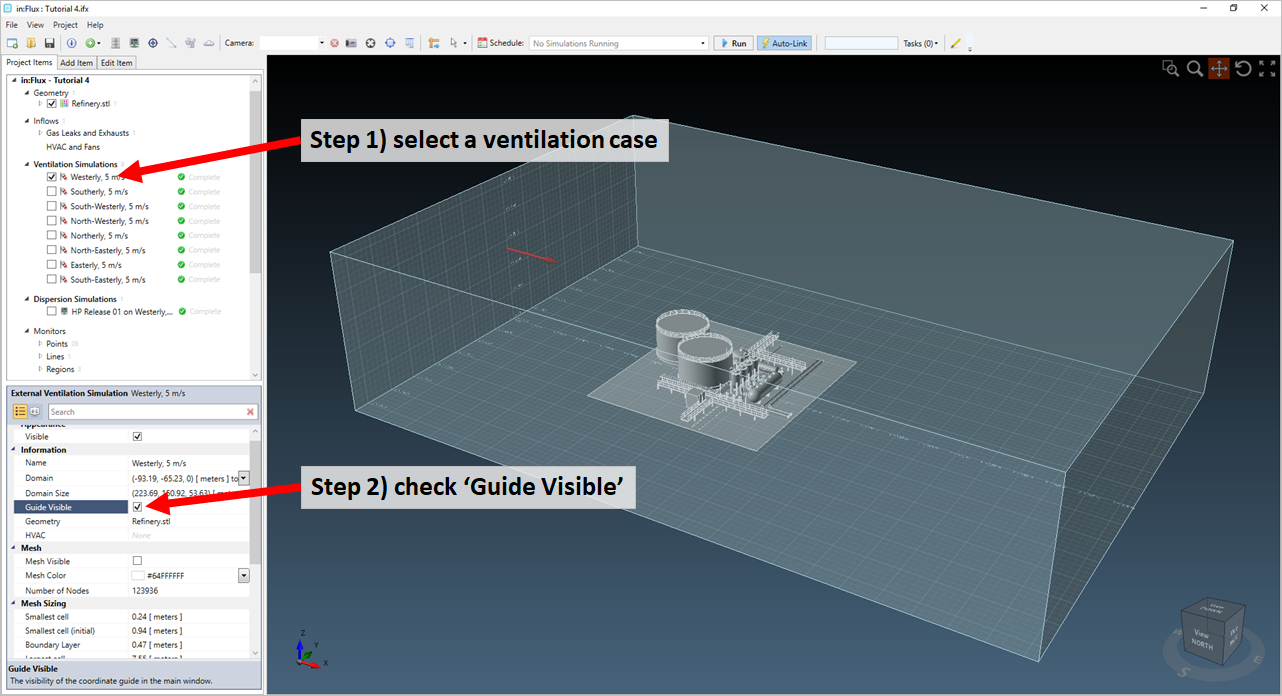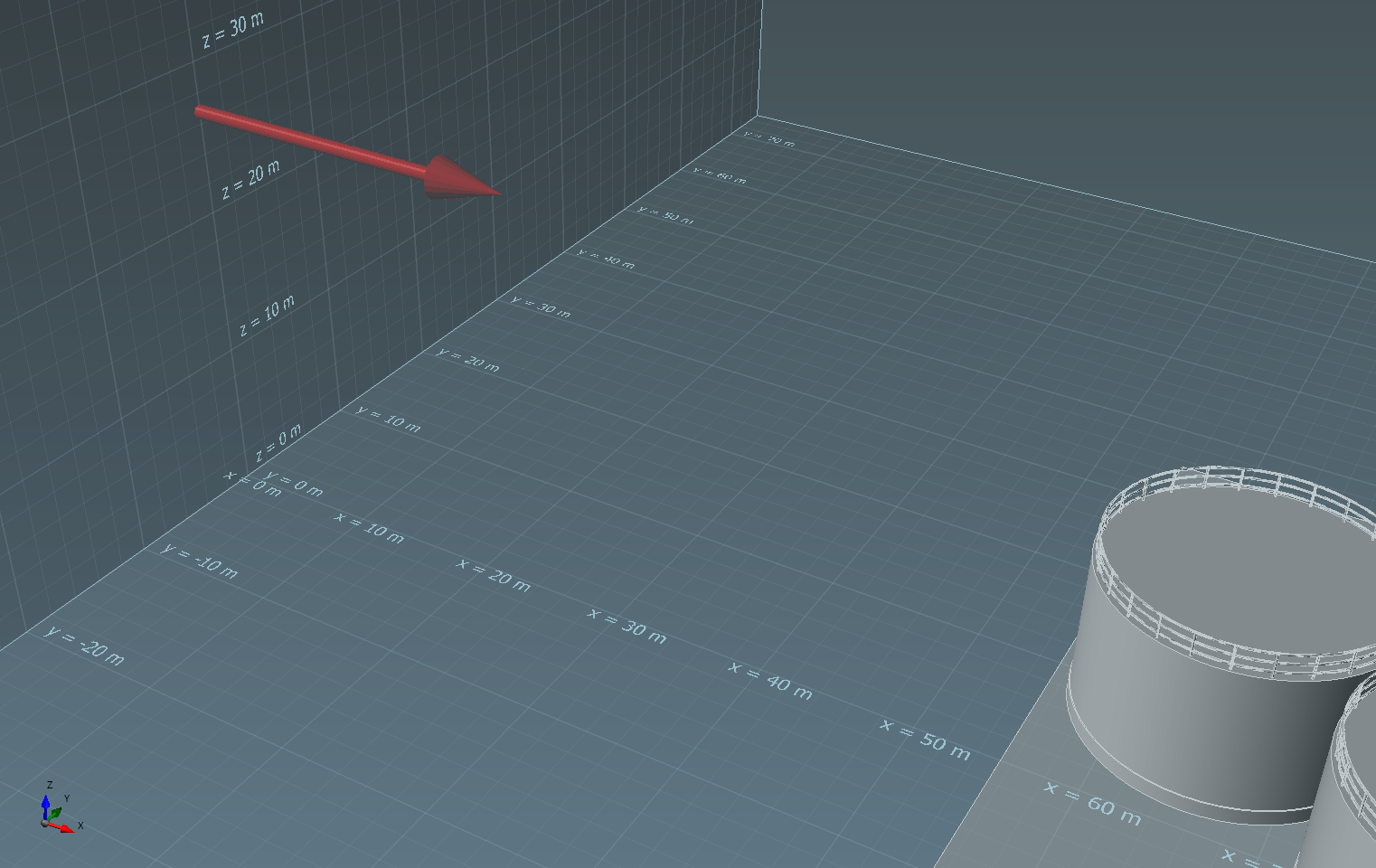## ‎ Visualization "Domain" Coordinate System

in:Flux has two coordinate systems: one relating to the Global set of coordinates and another based on the Domain of the ventilation simulation.

For visuals such as contours and vectors, the coordinate system is based on the Domain of the corresponding ventilation. You may view the coordinate system axis and grid by selecting the Guide Visible checkbox in the properties panel of each ventilation simulation, shown in the figure below for a westerly wind.Tutorial 6 - Figure 65 - turning on the 'guide' for the Westerly wind case of Tutorial 4

Zooming in you will see the grid and labels at every 10 meters.Tutorial 6 - Figure 66 - zoomed in view showing grid labels for domain guide

The Domain coordinate system is as follows for any simulation:

• X-axis being the streamwise direction

• Y-axis being the cross-stream direction

• Z-axis being in the vertical direction

Thus, for a vertical contour along the center line of the domain (in the same direction as the flow), select the ZX Plane with a offset of Y = 0 as in the below figure.Tutorial 6 - Figure 67 - Example vertical contour positioning based on the domain coordinate system